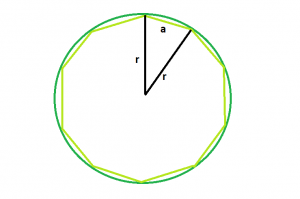Open in App
Not now

# Program to find the Circumcircle of any regular polygon

• Last Updated : 27 Aug, 2022

Given a n-sided polygon with side length a. The task is to find the area of the circumcircle of the polygon.

Examples:

```Input: n = 10, a = 3
Output: 1.99737```
```Input: n = 5, a = 6
Output: 3.02487```Approach: A regular n-gon divides the circle into n pieces, so the central angle of the triangle is a full circle divided by n: 360 deg/n
Applying the law of cosines for the three side lengths of the triangle, we get

```c2 = a2 + b2 - 2ab cos C
or, a2 = r2 + r2 - 2rr cos (360/n)
or, a2 = 2r2 - 2r2 cos (360/n)
or, c2 = r2 (2 - 2 cos (360/n))
so, a=r√(2-2cos(360/n))

Therefore,
r=a/√(2-2cos(360/n)) ```

Below is the implementation of the above approach:

## C++

 `// C++ Program to find the radius``// of the circumcircle of the given polygon` `#include ``using` `namespace` `std;` `// Function to find the radius``// of the circumcircle``float` `findRadiusOfcircumcircle(``float` `n, ``float` `a)``{` `    ``// these cannot be negative``    ``if` `(n < 0 || a < 0)``        ``return` `-1;` `    ``// Radius of the circumcircle``    ``float` `radius = a / ``sqrt``(2 - (2 * ``cos``(360 / n)));` `    ``// Return the radius``    ``return` `radius;``}` `// Driver code``int` `main()``{` `    ``float` `n = 5, a = 6;` `    ``// Find the radius of the circumcircle``    ``cout << findRadiusOfcircumcircle(n, a) << endl;` `    ``return` `0;``}`

## Java

 `// Java Program to find the radius``// of the circumcircle of the given polygon` `import` `java.io.*;` `class` `GFG {``  ` `// Function to find the radius``// of the circumcircle``static` `float` `findRadiusOfcircumcircle(``float` `n, ``float` `a)``{` `    ``// these cannot be negative``    ``if` `(n < ``0` `|| a < ``0``)``        ``return` `-``1``;` `    ``// Radius of the circumcircle``    ``float` `radius = (``float``)(a / Math.sqrt(``2` `- (``2` `* Math.cos(``360` `/ n))));` `    ``// Return the radius``    ``return` `radius;``}` `// Driver code` `    ``public` `static` `void` `main (String[] args) {``        ``float` `n = ``5``, a = ``6``;` `    ``// Find the radius of the circumcircle``    ``System.out.println( findRadiusOfcircumcircle(n, a)) ;` `    ``}``}``// This code is contributed``// by anuj_67..`

## Python 3

 `# Python3 Program to find the``# radius of the circumcircle``# of the given polygon` `# from math import all methods``from` `math ``import` `*` `# Function to find the radius``# of the circumcircle``def` `findRadiusOfcircumcircle(n, a) :` `    ``# these cannot be negative``    ``if` `n < ``0` `or` `a < ``0` `:``        ``return` `-``1` `    ``# Radius of the circumcircle``    ``radius ``=` `a ``/` `sqrt(``2` `-` `(``2` `*` `cos(``360` `/` `n)))` `    ``# Return the radius``    ``return` `radius` `# Driver code``if` `__name__ ``=``=` `"__main__"` `:` `    ``n , a ``=` `5``, ``6` `    ``# Find the radius of the circumcircle``    ``print``(``round``(findRadiusOfcircumcircle(n, a), ``5``))` `# This code is contributed``# by ANKITRAI1`

## C#

 `// C# Program to find the radius``// of the circumcircle of the given polygon``using` `System;` `class` `GFG``{` `// Function to find the radius``// of the circumcircle``static` `float` `findRadiusOfcircumcircle(``float` `n,``                                      ``float` `a)``{` `    ``// these cannot be negative``    ``if` `(n < 0 || a < 0)``        ``return` `-1;` `    ``// Radius of the circumcircle``    ``float` `radius = (``float``)(a / Math.Sqrt(2 -``                   ``(2 * Math.Cos(360 / n))));` `    ``// Return the radius``    ``return` `radius;``}` `// Driver code``public` `static` `void` `Main ()``{``    ``float` `n = 5, a = 6;` `    ``// Find the radius of the circumcircle``    ``Console.WriteLine(findRadiusOfcircumcircle(n, a));``}``}` `// This code is contributed``// by anuj_67`

## PHP

 ``

## Javascript

 ``

Output:

`3.02487`

Time Complexity : O(log(n)) because it is using inbuilt sqrt function
Auxiliary Space: O(1)

My Personal Notes arrow_drop_up